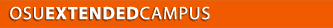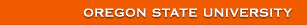Welcome Contact Getting Started Site Map Project 1 2 3 4 5 6 7 8 9 10 11

# Topic 5 - Dose Units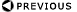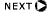### Dosimetry Overview

• Units
• Specific Gamma-Ray Emission
• Neutrons

### Introduction

• Dosimetry attempts to quantitatively relate specific radiation measurements to chemical/biological changes that could be produced
• Essential for quantifying biological changes as a function of radiation received
• For comparing experiments
• For other purposes
• Produces ionized and excited atoms and molecules
• Secondary electrons
• Produce additional ionizations and excitations
• Finally all energies are expended.
• Initial electronic transitions rapid (<10-15s)
• Represent the initial physical perturbations from which all effects evolve.
• So, ionization and energy absorption are the starting point for radiation dosimetry

### Quantities and Units

• Absorbed Dose
• Primary physical standard in dosimetry
• Defined as energy absorbed per unit mass from any kind of ionizing radiation in any target.
• SI Unit of absorbed dose,
• Called the gray (Gy)
• 1 Gy = 1 J kg-1
• Historical (older) term
• 1 Gy = 107 erg/103g = 104 erg/g = 100 rad
• Exposure
• Defined for X and gamma radiation
• In terms of ionization of air
• Old unit called roentgen (R)
• Initially defined in 1928, current definition is:
• 1 R º 2.58 x 10-4C kg-1 of air, exactly
• Applies only to electromagnetic radiation; the charge and mass refer only to air.

### Roentgen - original definition

• Amount of radiation that produced 1 esu of charge in 1 cm3 of air at STP
• 1 esu = 3.335 x 10-10C
•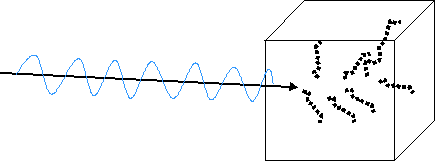• At STP air has a density of 0.001293 g cm-3
• 1 kg of air has a volume of 7.734 x 105 cm3
• 1 R = 3.335 x 10-10 C cm-3 of air
• How much energy is absorbed in air from 1R?

### Absorbed Dose and Exposure

• What is the absorbed dose in air when the exposure is 1 R?
• Need to know W for air
• 33.7 eV/ip = 33.7 J/C.
• 1 R º 2.58 x 10-4C/kg x 33.7 J/C = 8.8 x 10-3 J kg-1
• This equals 8.8 x 10-3 Gy (0.88 rad)
• Similar calculations show that 1R would produce a dose of 9.5 x10-3 Gy (=0.95 rad) in soft tissue.
• Why is there a difference between air and tissue???
• This is why one can say that 1 R ~ 1 rad in tissue.

### Exposure Measurement - Free Air Chamber

• Feasible to measure exposure at radiation energies between few keV and several MeV
• Definitive measure is by laboratory device known as free air chamber
• X-ray beam enters through a portal and interacts with cylindrical column of air defined by entry diaphragm
• Ions created in defined space are measured

### Parallel Plate Free-air Ionization Chambers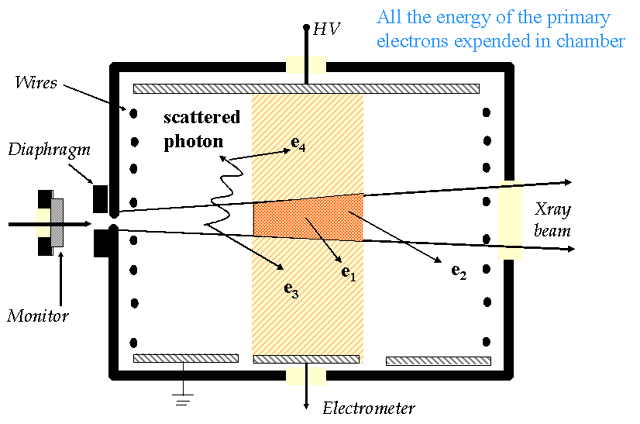### Free Air Chamber

• Photons enter chamber and interact with fixed quantity of air
• PE, CS
• Ions from air collected by plates
• Electric fields are kept perpendicular to plates by guard rings and guard wires
• Guard wires assure uniform potential drop across plates
• Field intensity is ~ 100 V/cm
• Collect ions prior to recombination
• Voltage low enough so no secondary ionizations
• Current flow is measured
• All energy of primary electrons must be deposited in sensitive volume of air for meter to work properly
• What if all primary electrons are not collected in sensitive volume?
• If equal number coming in from outside of sensitive volume as is going out: Electronic Equilibrium

### Electronic Equilibrium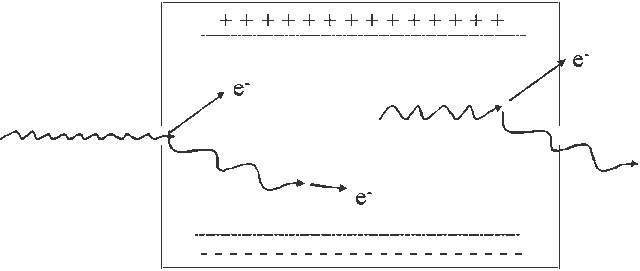• For every electron which escapes the sensitive volume, another electron of equal energy enters the sensitive volume and deposits energy in the detector
• A layer of air between entrance port of free air chamber and the sensitive volume can provide enough air so that electronic equilibrium is attained

### Free-Air Ionization Chamber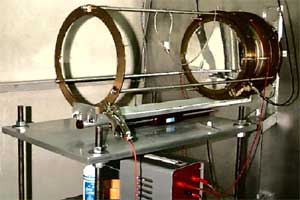### Wide-Angle Free Air Chamber (WAFAC)

• NIST primary standard for low-energy photon-emitting brachytherapy sources such as 125I.
• Variable volume, circular free-air chamber,
• Symmetrical about the beam axis, with lines of force parallel to the beam axis.
• 80 mm diam. Tungsten aperture (not visible at right) chamber used to define the beam.
• 3 electrodes: polarizing, middle, and collecting electrode (farthest from the source and left in the picture) about 150 mm in diameter.
• Chamber volume varied from ~75 cm3 to 804 cm3 by changing middle electrode from 11 mm to 152 mm.

### Measuring Exposure

• Free air chamber practical only as laboratory device
• Portable instrument needed

### Exposure Measurement: Air Wall Chamber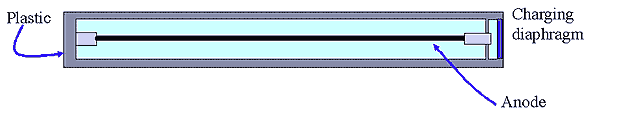• Practical alternative to free-air chamber
• Built as a capacitor
• Central anode, insulated from rest of chamber
• Given an initial charge
• When exposed to photons, 20 electrons neutralize charge & lower potential between anode and wall
• Change in potential difference is proportional to total ionization (and therefore exposure)
• Better for field use than the Free Air Chamber
• Simulates compressing air into a small volume by using air equivalent material
• X-ray absorption properties similar to that of air
• Walls must be thick enough to generate enough primary electrons
• Walls must be thin enough so that primary radiation is not shielded
• Ideal air wall chambers have only primary electrons ionizing the air in the sensitive volume
• Ideal wall thickness is almost energy independent over a range from 200 keV to almost 2 MeV (See figure 6.4)
• Greater than 3 MeV primary electrons have long range
• Impractical to build air wall chamber of sufficient size
• When walls are made thick enough to generate primary electrons, radiation is attenuated significantly
• Radiation intensity will no longer be constant
• Primary electrons not produced uniformly
• No electronic equilibrium

### Exposure-Dose Relationship

• Exposure
• measures charge produced in a mass of air
• C/kg
• Absorbed dose
• Measures energy absorbed per mass
• J/kg
• How to relate measurement in air to absorbed dose in something besides air?
• Energy absorption in air ¹ energy absorption in tissue
• Dose in air ¹ dose in tissue
• 1 R = 87.7 ergs/gair = 95 ergs/gtissue
• 1 rad = 100 ergs/gtissue
• For regulatory purposes, frequently 1 R is assumed to be equal to 1 rad
• Conversion can be done if required

### Exposure to Dose Conversion

• Equation 6.12
•  mm = Energy absorption coefficient for tissue
•  ma = Energy absorption coefficient for air
•  rm = Tissue density
•  ra = Air density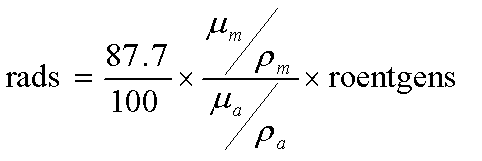### Bragg-Grey Theory

• How to measure absorbed dose?
• The best way would be calorimetry...but not very practical.  Instead, absorbed dose is measured by:
• measuring ionization
• use of correction factors
• calculating (approximating) dose
• This is done with BRAGG-GREY CAVITY THEORY

### Measurement of Absorbed Dose

• Bragg-Gray principle relates ionization measurements in a gas to absorbed dose in some material.
• Consider a gas in a walled enclosure irradiated by photons: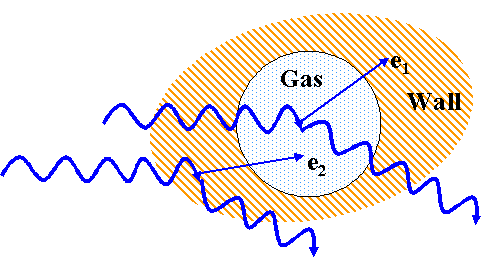• Photons interact in cavity and wall
• Chose wall material that has similar radiation absorption properties as tissue (e.g., Z)
• Cavity is very small
• (doesnt change angular and velocity distributions of 20 electrons)
• Electronic equilibrium exists in cavity
• (# e- stopping = # e- starting in cavity)
• requires wall thickness > range of 2o e
• Ionizations in the gas
• Can measure the charge liberated.
• If you know the energy required to ionize the gas,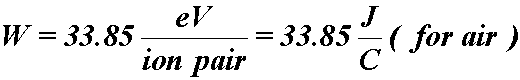• Then the dose to the gas is: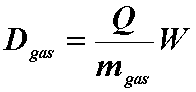• where,
• Q = coulombs of charge liberated
• W = average ionization energy for the gas
• m = kg of gas in the cavity
• Example: A cavity filled with (1 cm3) air at STP is exposed to a radiation field that liberates 3.336X10-10 C.  What is the dose to the air?
• At STP: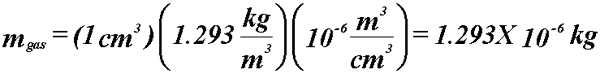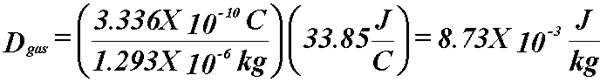• Know the dose to the gas.
• What about the dose to the medium surrounding it?
• Assume our cavity is really small...small enough that it does not disrupt the electron spectrum.
• Wall thickness must be as great as range as secondary charged particles (not too great to attenuate beam)
• Then energy absorbed per unit mass of wall is related to that absorbed per unit mass of gas by: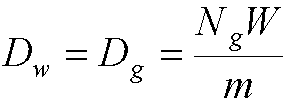• Note - this special case is where the wall and gas are the same type of material
• Dw is the dose to the wall
• Dg is the dose to the gas
• Nq is the number of ions produced in the gas
• W is eV required to produce and ion pair
• m is the mass of gas in the cavity
• If gas and wall dont have same atomic composition, a slight modification is required: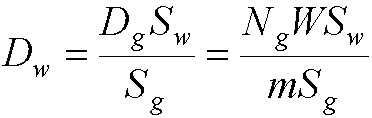• where Sg,w are the mass stopping powers of the wall and the gas
• the cavity and gas pressure must be small

### Example

• 1 cm3 of air in a block of carbon is exposed to 60Co γ
• Q=3X10-8 C is produced.
• What is the absorbed dose to the carbon?
•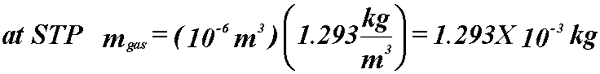• Mean mass stopping power ratio for 60Co γ in carbon relative to air (Table 6.1) = 1.009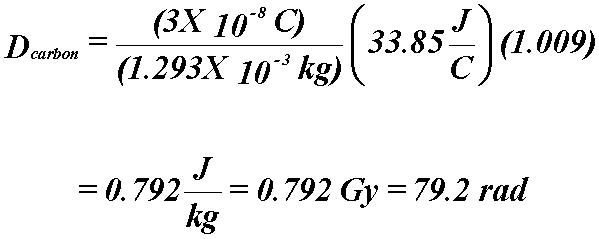• This equation allows us to measure the ionizations in a gas and relate it to dose to the medium.
• If neutrons are present, the wall must be at least as thick as the maximum energy range of any secondary charged recoil nuclei produced by the nuclear interactions.
• Chambers that meet these conditions can be used to measure absorbed dose to the medium
 Welcome Contact Getting Started Site Map Project 1 2 3 4 5 6 7 8 9 10 11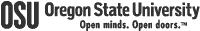College of Engineering OSU Extended Campus Local 541-737-9204 Fax 541-737-2734 4943 The Valley Library Corvallis, OR 97331-4504